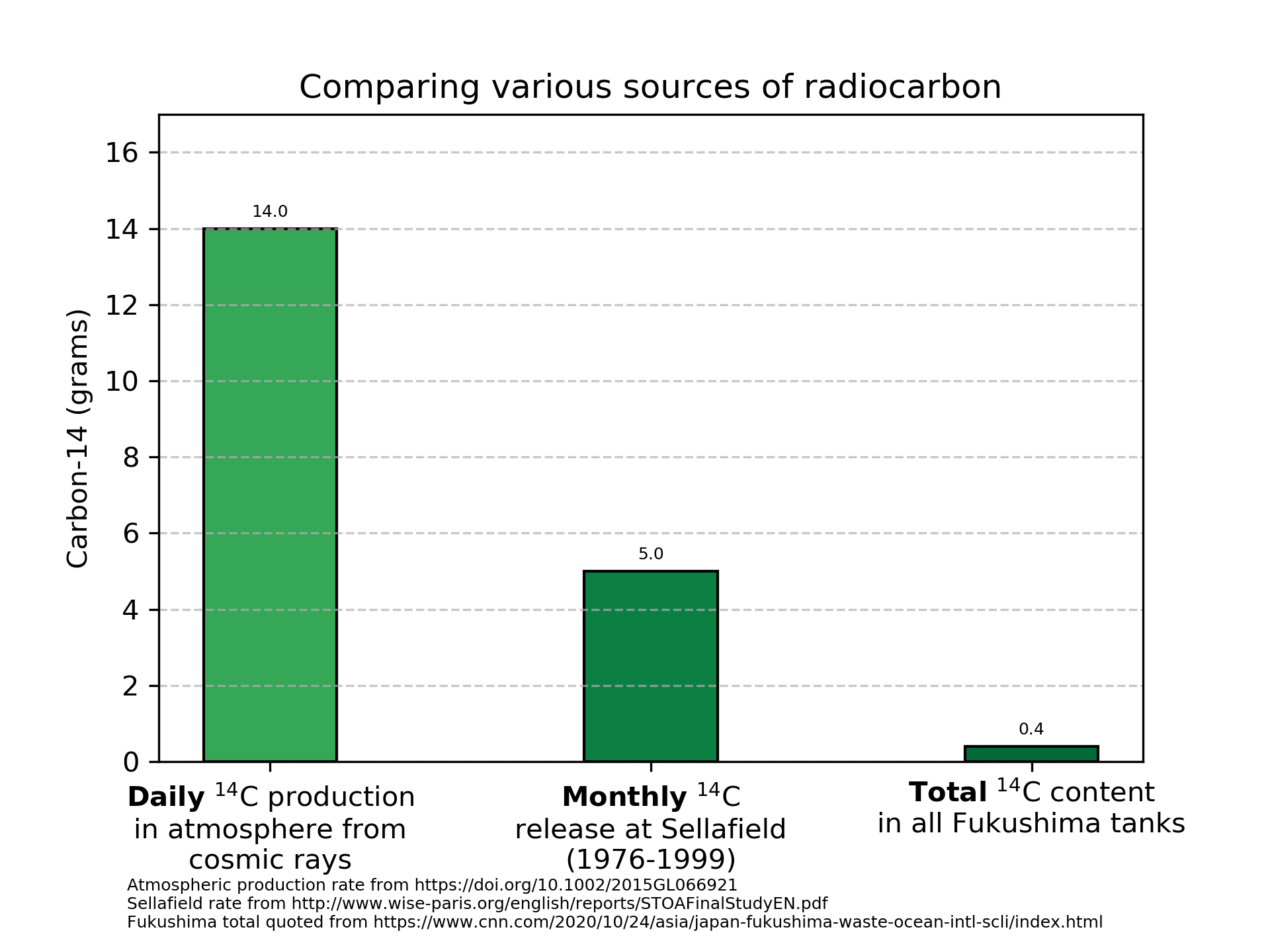# Greenpeace, CNN, The Hill miss context on radiocarbon release

##### Nick Touran, Ph.D. (nuclear engineering), 2020-10-25. Reading time: 3 minutes

On Saturday, Oct. 24th, CNN published an article with the following headline:Greenpeace is suggesting that the carbon-14 ($$^{14}$$C) in the tanks will be released in a way that is a serious threat to human health. Ionizing radiation can damage DNA, but life evolved in a naturally radioactive world. They fail to mention important contextual information that would help people assess the relative seriousness of this threat. This post fills in that contextual gap.## How much $$^{14}$$C are we talking about?

Greenpeace says there are 63.6 giga-Becquerel (GBq) of $$^{14}$$C in the tanks. How much is that? A Bq is a unit of radioactivity that is equivalent to 1 decay per second. To convert an activity (A) in Bq to number of atoms (N) of a radioactive substance, we use the formula:

$N = \frac{A}{\lambda}$

where $$\lambda$$ is the decay constant and is equal to $$\frac{ln(2)}{\tau_{1/2}}$$. For $$^{14}$$C the half-life $$\tau_{1/2}$$ is equal to 5700 years or 1.80e+11 seconds. Thus, 63.6 GBq is equal to

$\frac{63.6e9}{\frac{ln(2)}{1.8e11}} = 1.7e22 \text{ atoms of }^{14}C$

A mole of atoms is 6.022e23 atoms (Avogadro’s number), so this is 0.02755 moles. At ~14 grams/mole, this comes out to a grand total of 0.4 grams of $$^{14}$$C in all the tanks combined.

## Natural sources of $$^{14}$$C

You’ve heard of using carbon dating to figure out how old things are. This works because $$^{14}$$C is created continuously in our atmosphere when cosmic protons interact with our atmosphere. $$^{14}$$C has been made in our air constantly since well before humans evolved. How much is produced? Kanu, et al shows that 2.2(±0.6)e26 atoms of $$^{14}$$C are produced this way per year.

Again dividing by Avogadro’s number and multiplying by 14 grams/mol, we see that nature places 5100 grams of $$^{14}$$C per year into our atmosphere. This is equal to 14 grams per day.

Thus, nature makes the entire inventory of $$^{14}$$C in the tanks every ~40 minutes in the atmosphere.

But is atmospheric and ocean health effects comparable? Yes they are. The Sellafield report states quite clearly in §4.2.4.1:

Carbon-14 collective doses are similar whether released to atmosphere or sea.

## Historical releases of $$^{14}$$C

The Sellafield report referred to by Greenpeace shows that the Sellafield reprocessing plant released between 30 and 60 grams of $$^{14}$$C per year for at least 20 years. At this rate (again vastly higher than the total inventory at Fukushima released every year), it was assessed that the natural dose from $$^{14}$$C could nearly double for critical populations due to local concentrations. That could arguably be concerning for people sensitive to 2x their natural background dose.

But since the Fukushima release is so much smaller than this, and we didn’t see an alteration of human DNA from those larger releases, there is a good chance that the Fukushima discharges will not alter human DNA.